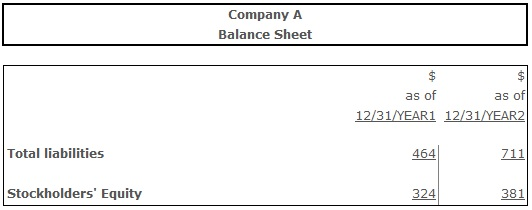# Debt to Equity Ratio

Debt to Equity Ratio – a ratio measuring the level of creditors’ protection in case of the firm’s insolvency by comparing its total debt with shareholders’ equity.

Normative for debt to equity ratio is the value ranging from 0,66 to 1,5. Identically to the debt ratio, values higher than normative indicate the high level of financial risks in a long-term perspective. Lower than normative values indicate the incomplete usage of company's potential, since the credit capital could be used for different kinds of firm's activities.

## Formula(s):

Debt to Equity Ratio = Total Liabilities ÷ Shareholders’ Equity

## Example:Debt to Equity Ratio (Year 1) = 464 ÷ 324 = 1,43

Debt to Equity Ratio (Year 2) = 711 ÷ 381 = 1,86

The increase of the debt to equity ratio from 1,43 in year 1 to 1,86 in year 2 indicates that firm has most likely been financing its growth with debt in order to generate more earnings. At the same time, firm’s long-term debt-paying ability has decreased. In other words, for every dollar of equity company used to have 1 dollar and 43 cents of liabilities in year 1, while in year 2 the amount of liabilities per dollar of equity increased to 1 dollard and 86 cents.

## Conclusion:

Debt to equity ratio reflects the relationships between the stockholders' equity and total liabilities of the company. Efficient usage of the debt (in case operating earnings are much higher than charges associated with debt) leads to the situation, when shareholders receive their returns through financial leverage effect. For a firm preferable is the situation, when debt to equity ratio lies within the normative range from 0,66 to 1,5.